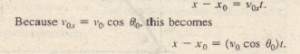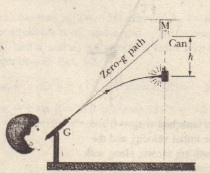# Projectile Motion Analyzed

Projectile Motion Analyzed

Now we are ready to analyze projectile motion, horizontally and vertically.

The Horizontal Motion

Because there is no acceleration in the horizontal direction, the horizontal component  of the projectile’s velocity remains unchanged from its initial value  throughout the motion as demonstrated in . At any time  the projectile’s horizontal displacement from an initial position  is given by which we write asThe Vertical Motion

The vertical motion is the motion we discussed in for a particle in free fall. Most important is that the acceleration is constant. Thus, the equations of provided we substitute -g for a and switch to y notation.The projectile ball always hitthe falling can. Each falls a distance hfrom where it would be were there no.free-fall acceleration.

and its magnitude steadily decreases to zero, which marks the maximum height of the path. The vertical velocity component then reverses direction, and its magnitude becomes larger with time.

The Equation of the Path

We can find the equation of the projectile’s path (its trajectory) by eliminating t between for t and substituting into, we obtain, after a little rearrangement.This is the equation of the path shown in . In deriving it, for simplicity respectively. Because constants of the form which a and b are constants. This is the equation of a parabola, so the path is parabolic.

The Horizontal Range

The horizontal range R of the projectile as  shows, is the horizontal distance the projectile has traveled when it returns to its initial (launch) height. To find range R, let us put x and y, obtainingCaution: This equation does not give the horizontal distance traveled by a projectile when the final height is not the launch height.The vertical component of thisskateboarder’s velocity is changing,but not the horizontal component,which matches the skateboard’s velocity,As a result, the skateboard staysunderneath him, allowing him to landon it.

The horizontal range R is maximum for a launch angle of 45°.

The Effects Of the Air

We have  assumed that the air through which the projectile moves has no effect on its motion. However, in many situations, the disagreement between our calculations and the actual motion of the projectile can be large because the air resists (or opposes) the motion,for example, shows two paths for a fly ball that leaves the